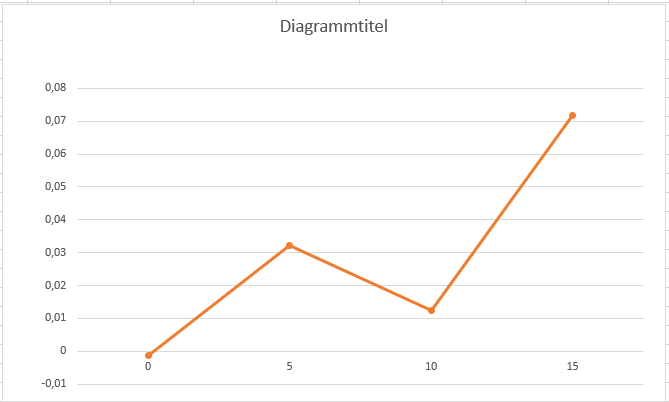# Calculation of coefficientes for simulatio, Software in the loop

Hi,

As part of my bachelor’s thesis I need to simulate on gazebo a Glider modelled in a CAD software based from a real small RC Glider.

I am trying to do what this tutorial explains.

Here is where Simscale comes in play:
To get a linearisation for the alpha/lift curve, alpha/drag curve and the alpha/moment curve what I am doing is rotate the STLs at different angles of attack ( say 0, 2.5, 5, 7.5, 10, 12.5) and then calculate the coefficients for each STL.
This process is tedious since i gotta do this for 5 control surfaces and the fuselage. But i guess there isnt a way around ¯(°_o)/¯ ?
After that, I think it should be enough to do a linear regression to get the ratio of each linearisation.

This is my project.

My questions are:

1. Am I using the right turbulence model? Im using incompressible LES Spalart-Allmaras with transient time dependecy (the only option available for LES Spalart-Allmaras). The velocity of the flow is relatively slow, 6-7 m/s. I was also thinking that maybe the laminar turbulence model would suit for the first angles, up to 9/10 deg, after that, I guess I should use a different turbulence model, since the laminar flow starts to break at higher angles, correct?

2. I ve tried to calculate the coefficients for the elevator, as seen in the project. Those are the plots.

AoA 0:

AoA 5:

**AoA 10: **

AoA 15:

I dont know how to validate the data, they seem to coverge but I dont know if that is a valid argument to validate the data.
How could I validate it?

Here are the Residuals for the AoA 15.

The p value converges againt 0.5, what does this mean?

1. Here is a plot on excel of the coefficient of lift. (There is not a linear regression, it is only a connecting the points - plot)Why does it go back up? Is there a convergence problem for the simulation on the configuration AoA 15?
Or maybe the error is on the simulation for the configuration AoA 10 and there isnt a break of the laminar flow yet?
I know that I should do more simulations for several AoA configurations to get a better linear regression, but I dont want to use many computing hours while i dont know if the configuration of the CFD simulation is right.

Please, help meAlejandro.

Hey there Alejandro and thanks a lot for that detailed post, dope project I have to sayWhat I immediately noticed is that your domain is IMHO too small to reach proper solutions, and I would most likely use another turbulence model for that case and use resources that you can find online to see which turbulence models turned out to give you “good” results (example for Naca profiles: 2D NACA 0012 Airfoil Validation for Turbulence Model Numerical Analysis) - maybe the @cfd_squad can add their two cents here as well.

Best,

Jousef

1 Like

Alejandro,

Here are a few resources on Turbulence model choice if you haven’t seen these already.

Lecture 6 Turbulence Modeling Introduction to Introduction to ANSYS FLUENT

Fluent-Intro_15.0_L07_Turbulence.pdf - Lecture 7 Turbulence…

Dan

3 Likes